# How to Use the Barplot() Function in R

The barplot() function in R is “used to create a bar chart with vertical or horizontal bars”. The bars can have different colors, and their heights can be based on a vector or matrix of numeric values.

### Syntax

``barplot(height, xlab, ylab, main, names.arg, col)``

### Parameters

1. height: A vector or matrix containing numeric values used in a bar chart.
2. xlab: It is the label for the x-axis.
3. ylab: It is the label for the y-axis.
4. main: It is the title of the bar chart.
5. names.arg: It is a vector of names appearing under each bar.
6. col: It is used to give colors to the bars in the graph.

There are lots of other parameters, but you will mostly use these.

## How to use the barplot() function

To create a simple barplot, use the input vector and the name of each bar.

``````data <- c(11, 18, 19, 21, 46)

barplot(data)``````

Output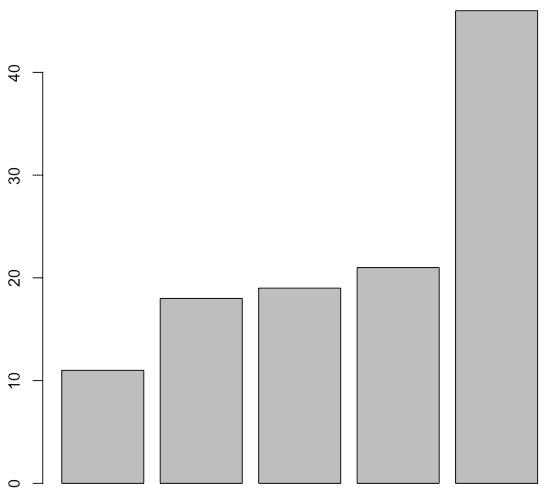In this example, the height is a vector, or we can say our data, so the values determine the heights of the bars in the plot.

This bar chart has not mentioned any x-label, y-label, main title, color, or other properties. But we can define it.

## Bar Plot Labels, Title, and Colors

To add a title in the bar plot, use the main parameter.

To add the colors in the bar, use the col parameter.

To define an x-axis name, use the xlab and the y-axis name, and use the ylab parameters.

``````data <- c(11, 18, 19, 21, 46)

barplot(data, main = "Enroll Chart",
xlab="x-axis", ylab="y-axis",
col="skyblue")``````

Output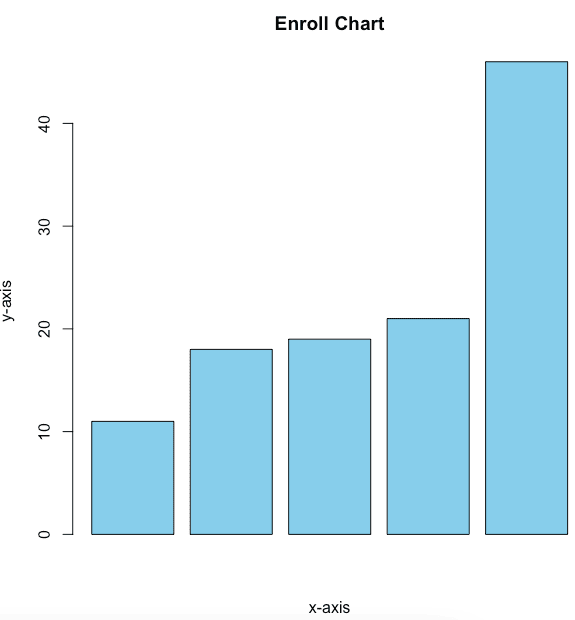## How to Create a Barplot with two vectors in R

To create a barplot with two vectors in R, you can use one vector for the x-axis and one for the y-axis or the matrix with two rows or columns representing the two vectors.

``````price <- c(580, 1040, 1980, 2810, 3125)
stocks <- c("Happiest", "TechMahindra", "Mindtree", "LTTS", "TCS")

barplot(price, names.arg= stocks,
main = "Stocks Pile",col="skyblue"
xlab = "Stocks Name", ylab = "Stocks Price")``````

Output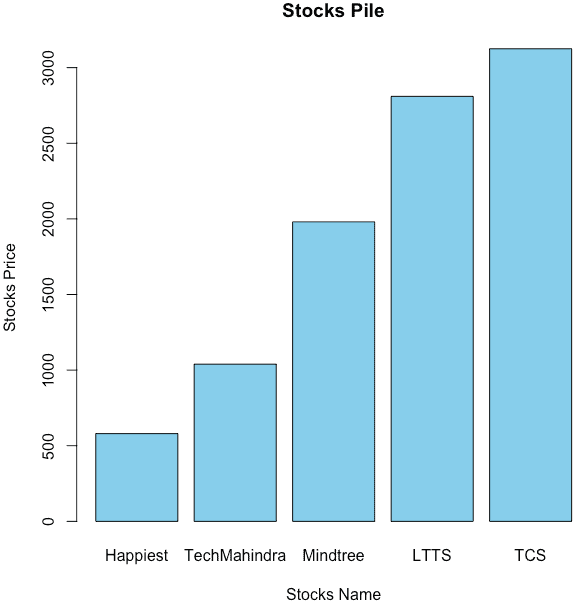## How to create a Horizontal barplot from a dataset using barplot()

To create a horizontal barplot from a dataset in R, you can use the “barplot()” function with the horiz=TRUE argument.

``````counts <- table(mtcars\$gear)
barplot(counts, names.arg=c("3 Gears", "4 Gears", "5 Gears"),
main="Car Distribution",
horiz=TRUE, col="skyblue")``````

Output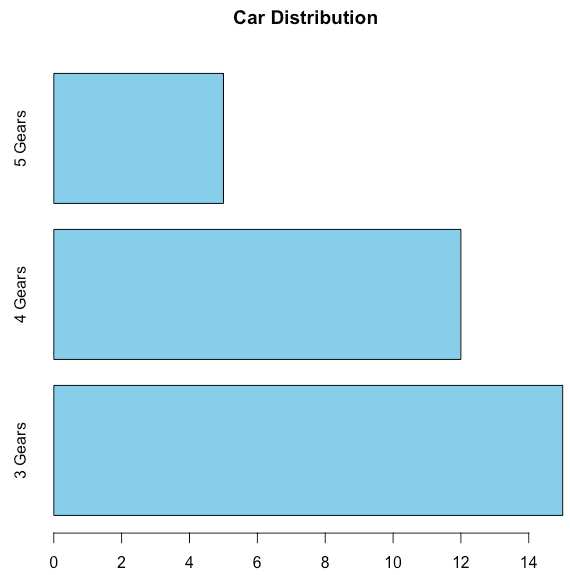## What is Grouped and Stacked Bar Chart in R

The Stacked Bar Chart in R Programming is handy in comparing the data visually. We can create a group and stack barplot using a matrix as input values. More than two variables are represented as a matrix.

### Stacked bar plot

``````counts <- table(mtcars\$vs, mtcars\$gear)
barplot(counts, main = "Car Distribution Channel",
xlab = "Number of Gears", col = c("skyblue", "red"),
legend = rownames(counts))``````

#### Output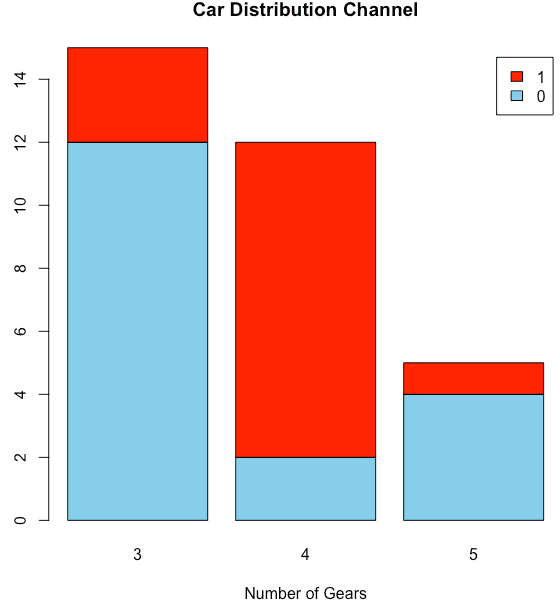### Grouped Bar Plot in R

``````counts <- table(mtcars\$vs, mtcars\$gear)
barplot(counts, main = "Car Distribution Channel",
xlab = "Number of Gears", col = c("skyblue", "red"),
legend = rownames(counts), beside = TRUE)``````

#### Output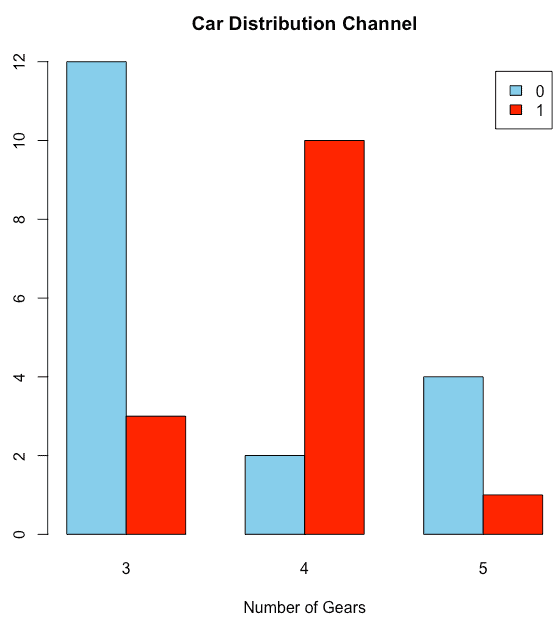To add a legend in the barplot, pass the legend argument with the values if you specify legend.text = TRUE, legend values are automatically assigned.

### 1 thought on “How to Use the Barplot() Function in R”

1.Nicely done and written my friend.
I’ve just started writing a blog myself very recently and realized that many bloggers simplyy rehash old ideas
but add very likttle of value. It’s fantastic to read a beneficial article of
some actual value too myself and your other
followers.
It is actually going down on my list off things I need too emulate as
a new blogger. Reaader engagement and material value are king.

Many terrific suggestions; you’ve definitely got on my list of writers
to follow!

Continue the excellent work!
Alll the best,
Pauline